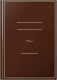# Controlled Markov Processes and Viscosity SolutionsSpringer, 2006 - Automatic control - 428 pages
This book is intended as an introduction to optimal stochastic control for continuous time Markov processes and to the theory of viscosity solutions. The authors approach stochastic control problems by the method of dynamic programming. The text provides an introduction to dynamic programming for deterministic optimal control problems, as well as to the corresponding theory of viscosity solutions. A new Chapter X gives an introduction to the role of stochastic optimal control in portfolio optimization and in pricing derivatives in incomplete markets. Chapter VI of the First Edition has been completely rewritten, to emphasize the relationships between logarithmic transformations and risk sensitivity. A new Chapter XI gives a concise introduction to two-controller, zero-sum differential games. Also covered are controlled Markov diffusions and viscosity solutions of Hamilton-Jacobi-Bellman equations. The authors have tried, through illustrative examples and selective material, to connect stochastic control theory with other mathematical areas (e.g. large deviations theory) and with applications to engineering, physics, management, and finance.; In this Second Edition, new material on applications to mathematical finance has been added. Concise introductions to risk-sensitive control theory, nonlinear H-infinity control and differential games are also included.

### What people are saying -Write a review

We haven't found any reviews in the usual places.